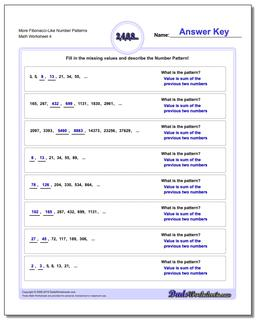# Math Worksheets: Number Patterns: Number Patterns: More Fibonacci-Like Number Patterns (Fourth Worksheet)## More Fibonacci-Like Number Patterns (Fourth Worksheet)

PropertyValue
DescriptionMore Fibonacci-Like Number Patterns: Fibonacci and Fibonacci-like number pattern problems, where the next values in a sequence are dependent on prior values. (Fourth Worksheet)
Resource TypeWorksheet